# Geometric Shapes

Go back to  'Geometry'

 1 Geometrical Shapes: Definition 2 List of Geometrical Shapes 3 Two Dimensional Shapes 4 Three Dimensional Shapes 5 Solved Examples on Shapes 6 Practice Questions on Shapes 7 Challenging Questions on shapes 8 Maths Olympiad Sample Papers 9 Frequently Asked Questions (FAQs)

We at Cuemath believe that Math is a life skill. Our Math Experts focus on the “Why” behind the “What.” Students can explore from a huge range of interactive worksheets, visuals, simulations, practice tests, and more to understand a concept in depth.

Book a FREE trial class today! and experience Cuemath’s LIVE Online Class with your child.

## Geometrical Shapes: Definition

Geometrical shapes are the figures or objects that we see around us everywhere.

These different shapes of geometry are made up of lines, curves, angles and surfaces.

The shapes in geometry can be either open or closely bound.

### Open Shapes

Lines or curves that do not connect at any one point are called open shapes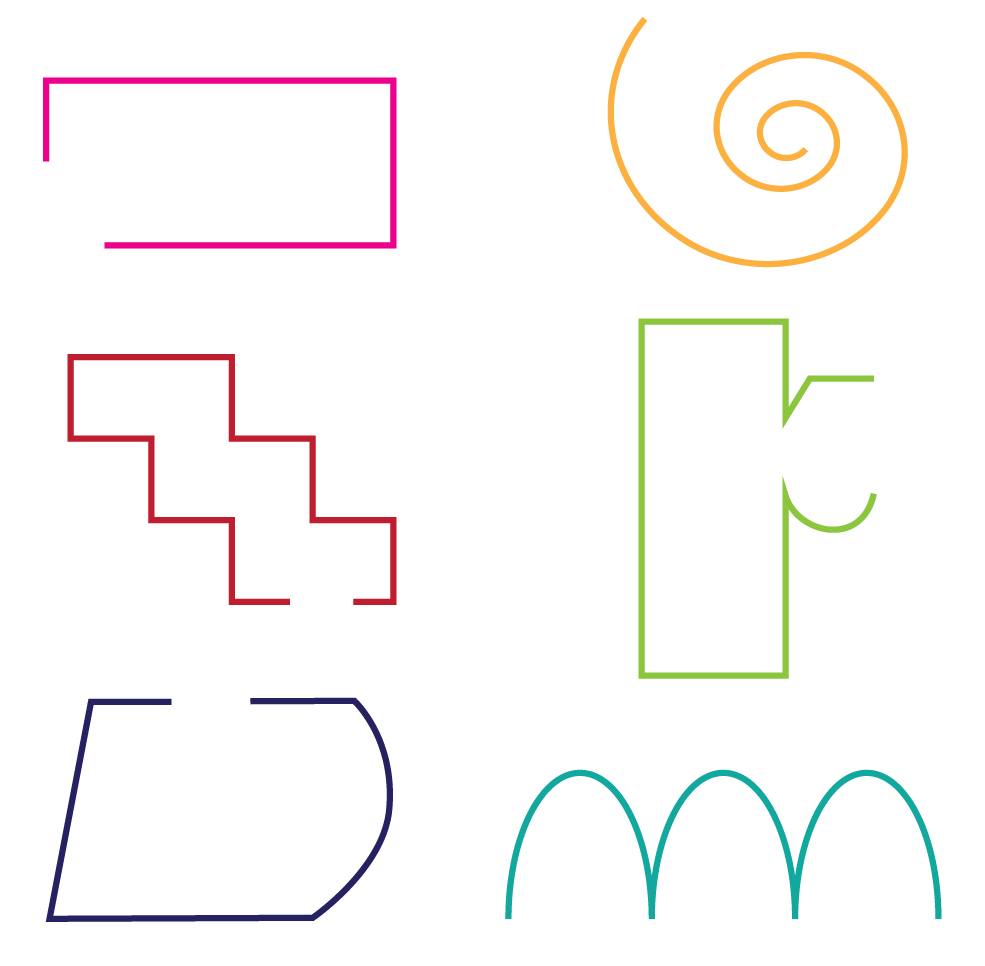### Closed Shapes

In closed shapes, all the lines and curves are connected with each other.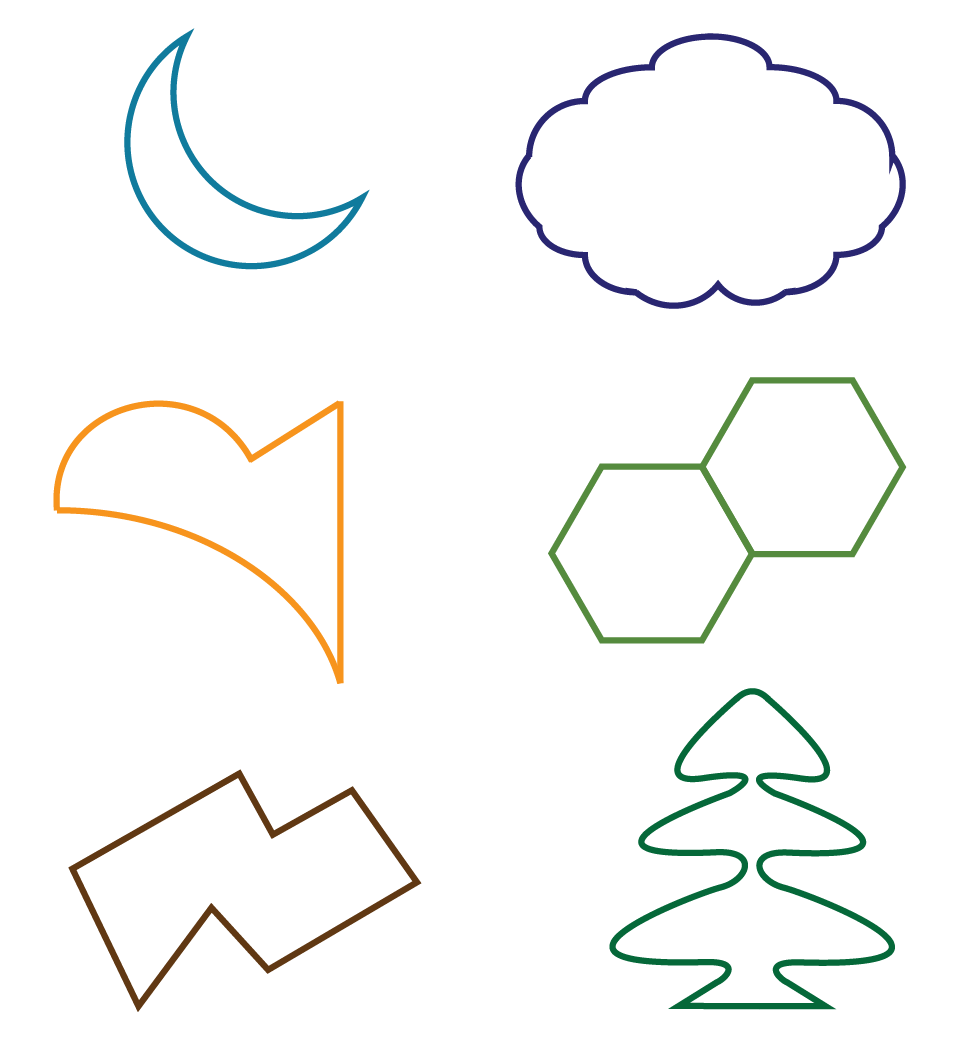There are many different types of shapes in Maths.

We will learn about them shortly.

Now, try to draw some fun shapes using various colours.

Identify if these Math shapes are open or closed.

## List of Geometric Shapes

There are many geometrical shapes we see around us every day.

Mathematical shapes can be two dimensional and three dimensional.

Two-dimensional shapes include circles, triangles, rectangles, squares, stars, hearts, ovals, and so on.

These shapes have only two dimensions or parameters.

Three-dimensional shapes include cones, spheres, cubes, cuboids, cylinders, etc.

These shapes have three dimensions and many faces.

Let us look briefly into two dimensional and three dimensional shapes.

## Two-Dimensional shapes

A 2D shape in maths consists of only two dimensions such as length and breadth.

It consists of a flat surface.

Some of the common 2D shapes in Maths are listed below.

### 1. Circle

A circle is a closed two-dimensional figure which is round in shape.

A circle does not have any sides or corners.

Can you spot any figure with the shape of a circle around you?

Here are some familiar objects that have the shape of a circle.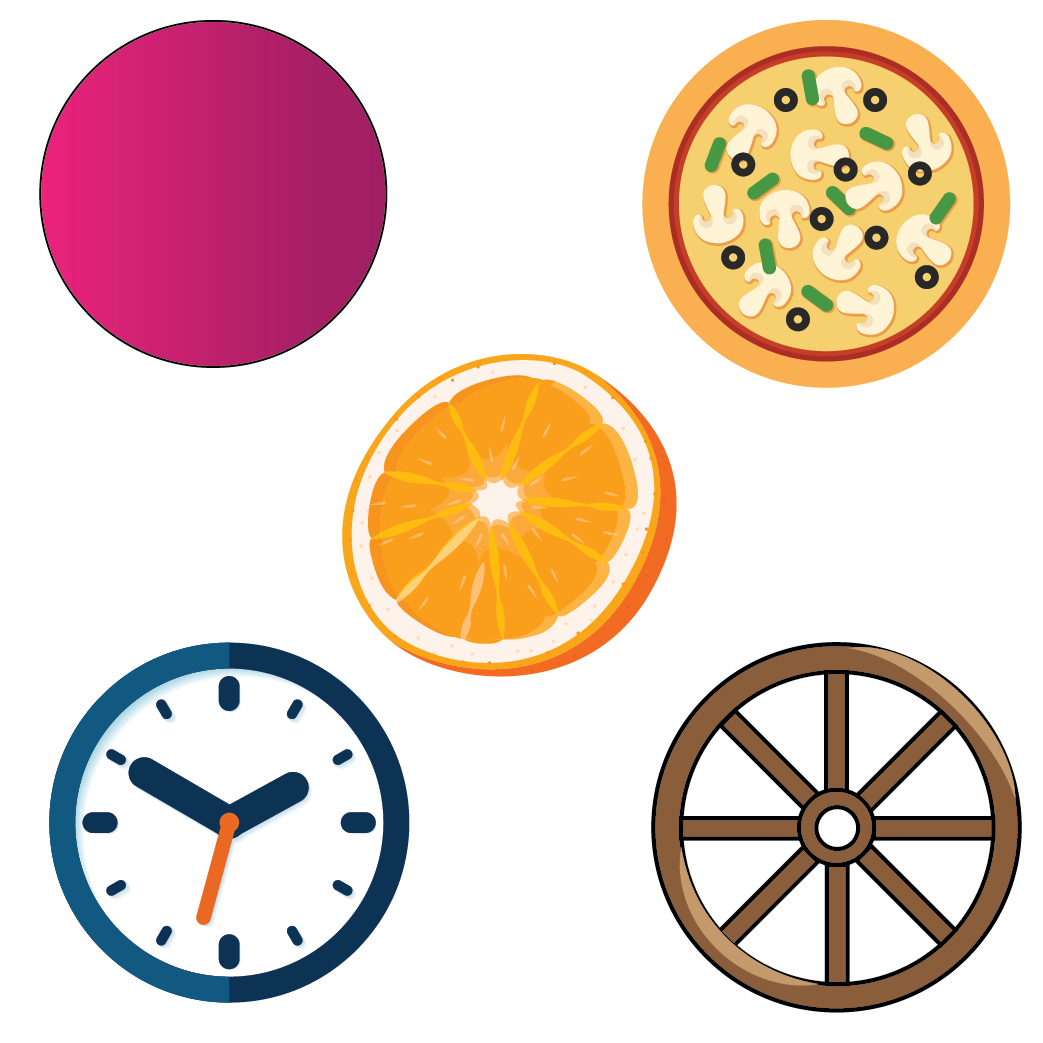### 2. Square

A square is a two-dimensional shape that consists of four sides which are all equal.

Can you think of any figure or object that is square in shape?

Do you play chess or carrom?

Imagine the shapes of a chessboard and a carrom board.

You can see that all the four sides of these boards are equal.

Thus, they are squares.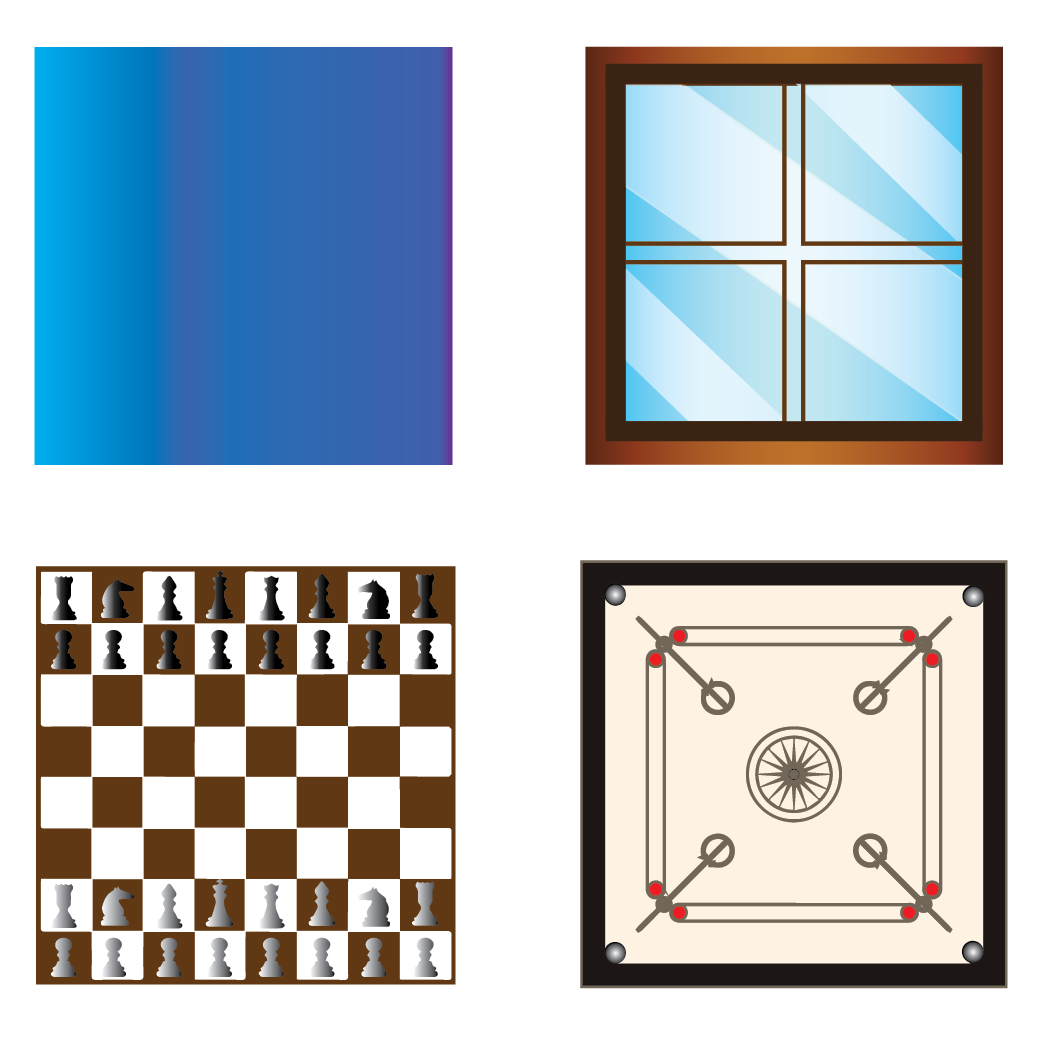### 3. Triangle

A triangle is a closed two-dimensional shape with three sides.

Do you like watermelon?

Most of us like it.

We cut the watermelon into slices.

Have you ever observed the shape of a slice of watermelon?

It is triangular in shape.

Have you observed the shape of a slice of pizza?

Do you think it is triangular in shape?

Yes, you are right, it is triangular in shape.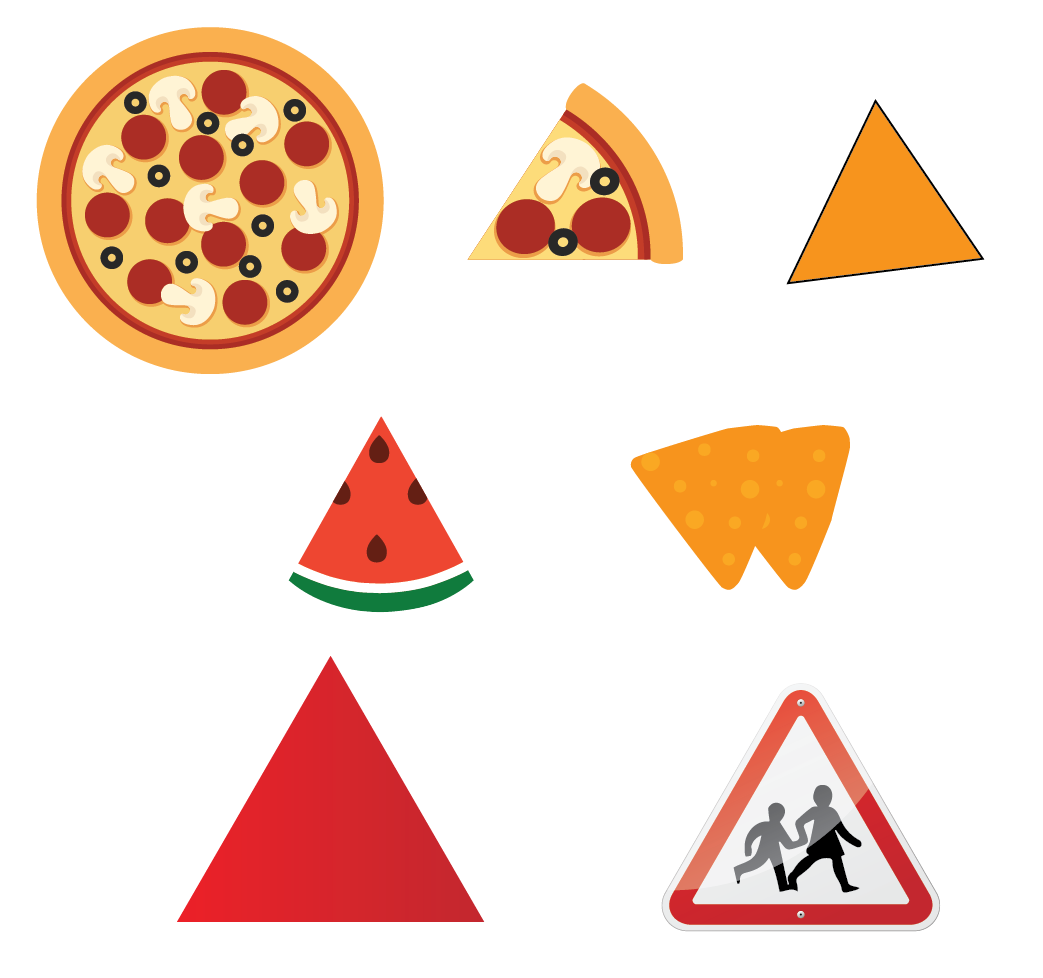### 4. Rectangle

A rectangle is a four-sided, two-dimensional shape in which the length of the opposite sides are equal.

A square is also a type of rectangle.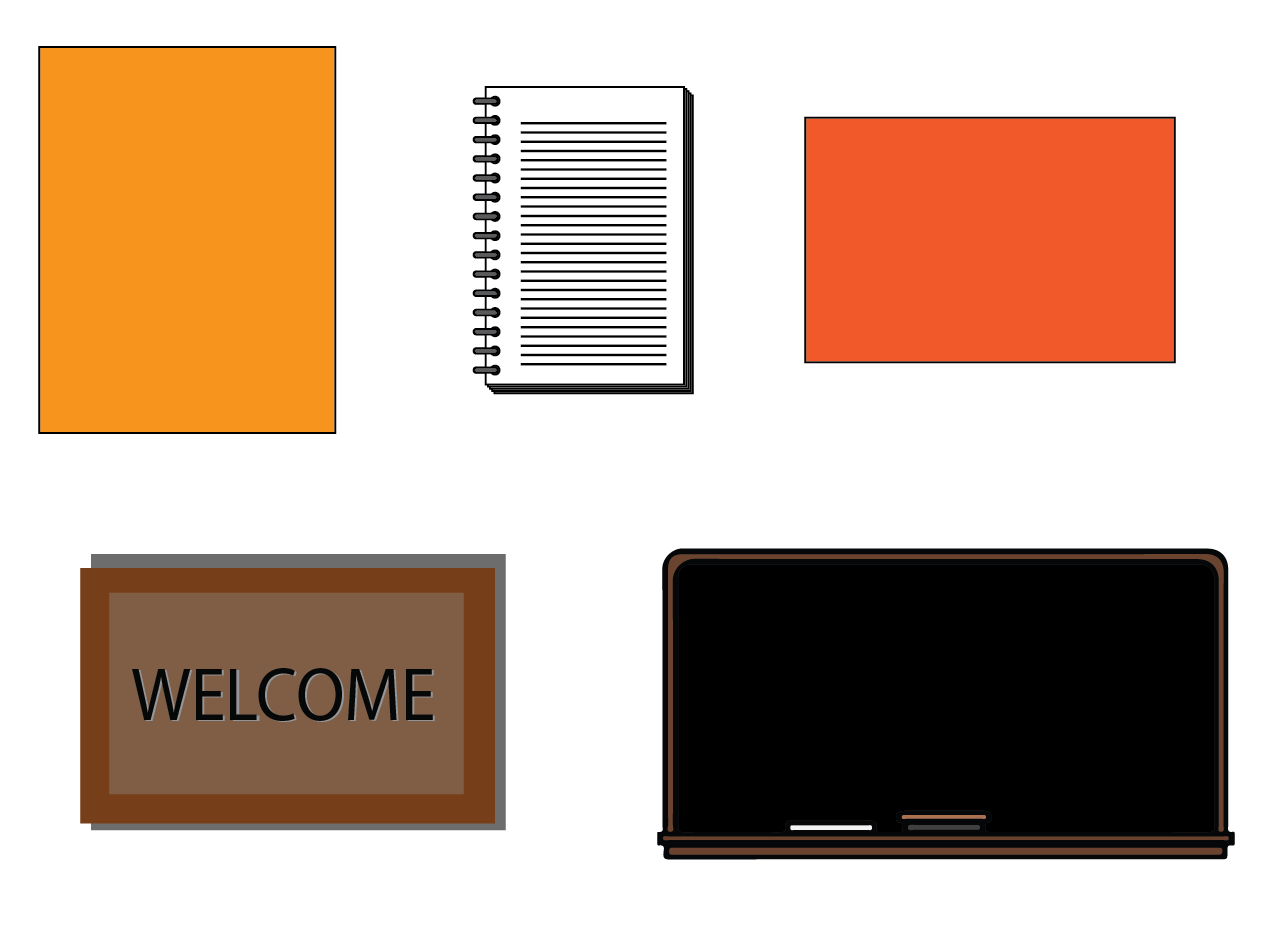Rectangular shape can have two variations in their forms: either the length or the breadth can be greater.

Now look around and identify objects that are rectangular in shape.

Observe your computer or laptop monitor.

What shape does it have?

Yes, it is a rectangle!

### 5. Oval

An oval is similar to a circle but its shape is slightly elongated in nature.

An oval also does not have any sides or corners.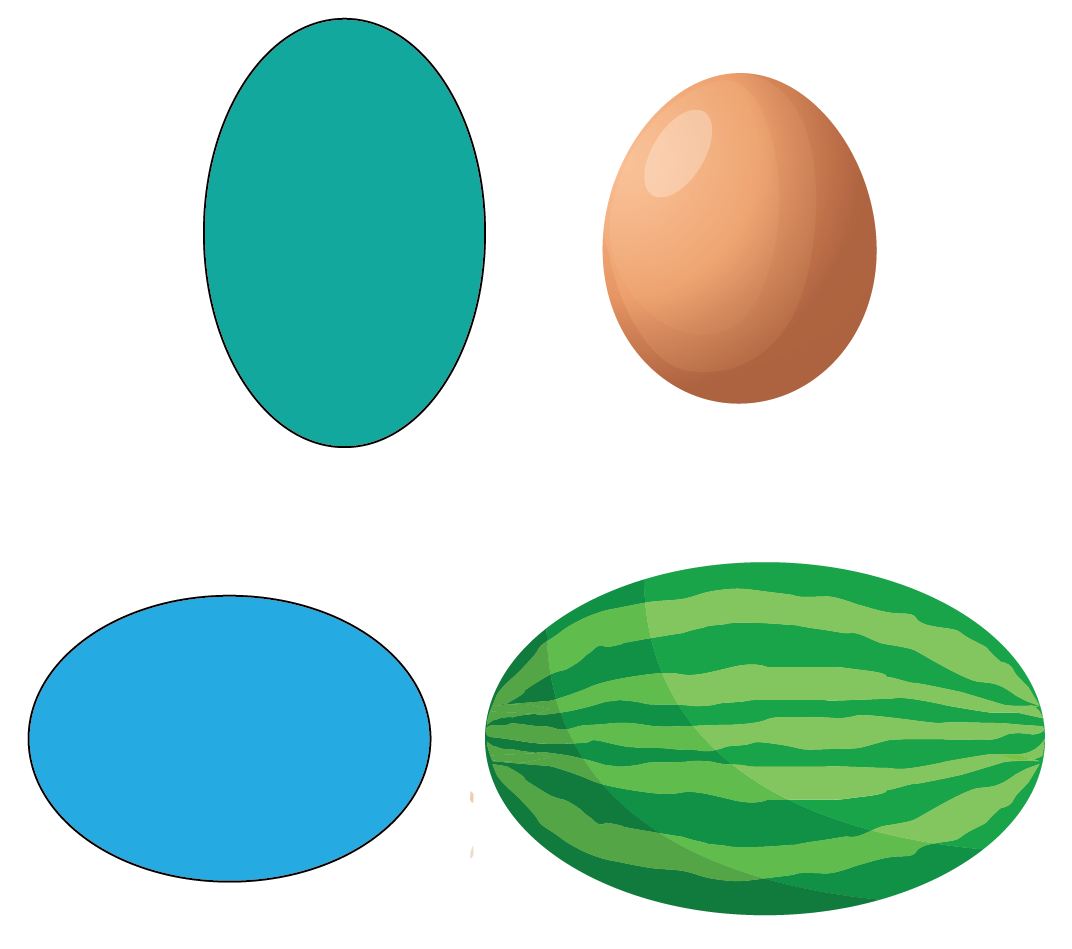Similar to the shapes described above, there are several two-dimensional shapes in geometry.

Now, let's summarize all the 2D shapes.

###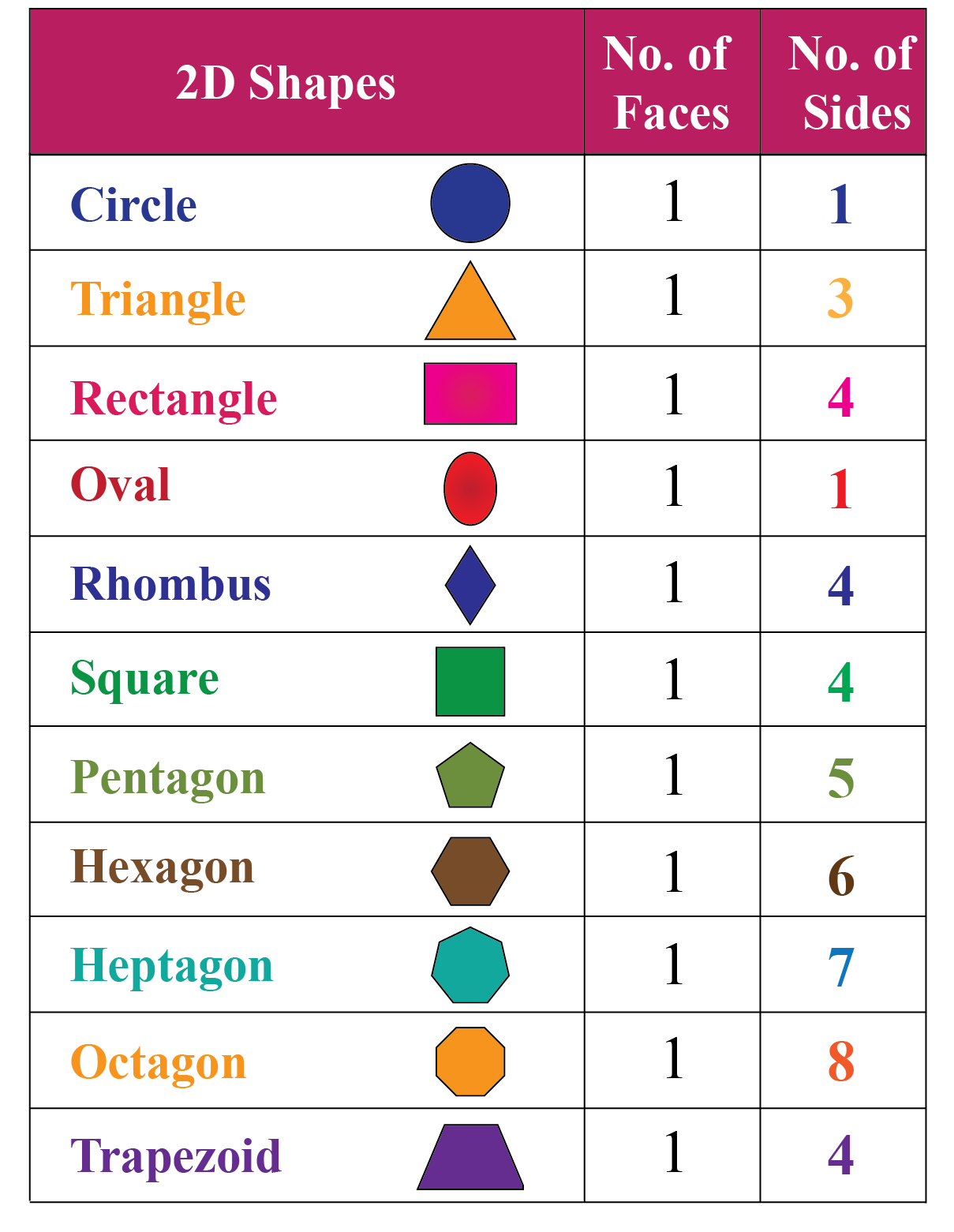Look around you and identify more shapes with two dimensions.Important Notes

Two-dimensional shapes that have four sides (square, rectangle, kite, trapezoid) are called quadrilaterals.

In general, any shape with four sides is considered a quadrilateral shape.

Look at the following pictures and identify the shape of each object.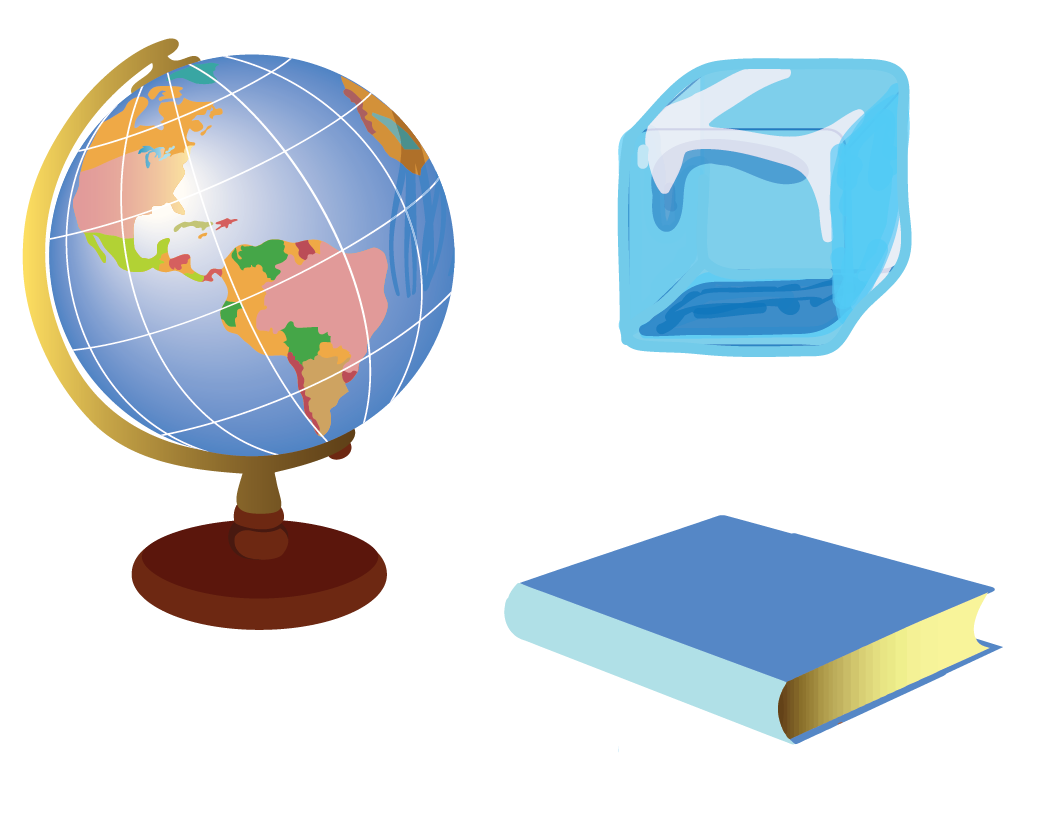Are these two-dimensional shapes?

No, these shapes have length, breadth, and some thickness.

Hence, these objects have three dimensions.

## Three-Dimensional Shapes

A 3D shape is a solid figure that consists of three dimensions such as length, breadth, and height.

Three-dimensional shapes have some thickness or depth.

Mathematical shapes are present everywhere and we see many different shapes around us.

Everything we see has some shape.

Consider this example of a globe.

This is the globe of our planet, Earth.

Can you guess what is the shape of our planet?

It is a sphere.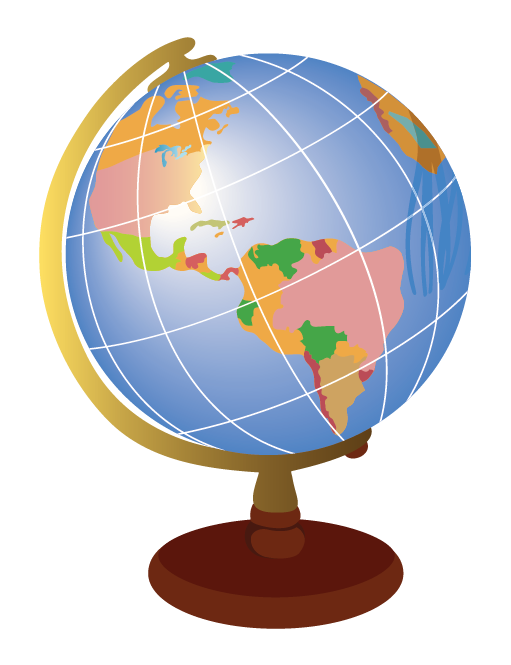### Sphere

A sphere is a three-dimensional shape that rotates in a circle.

A sphere does not have any sides or corners.

Have you observed a football or a basketball?

These balls are examples of spheres.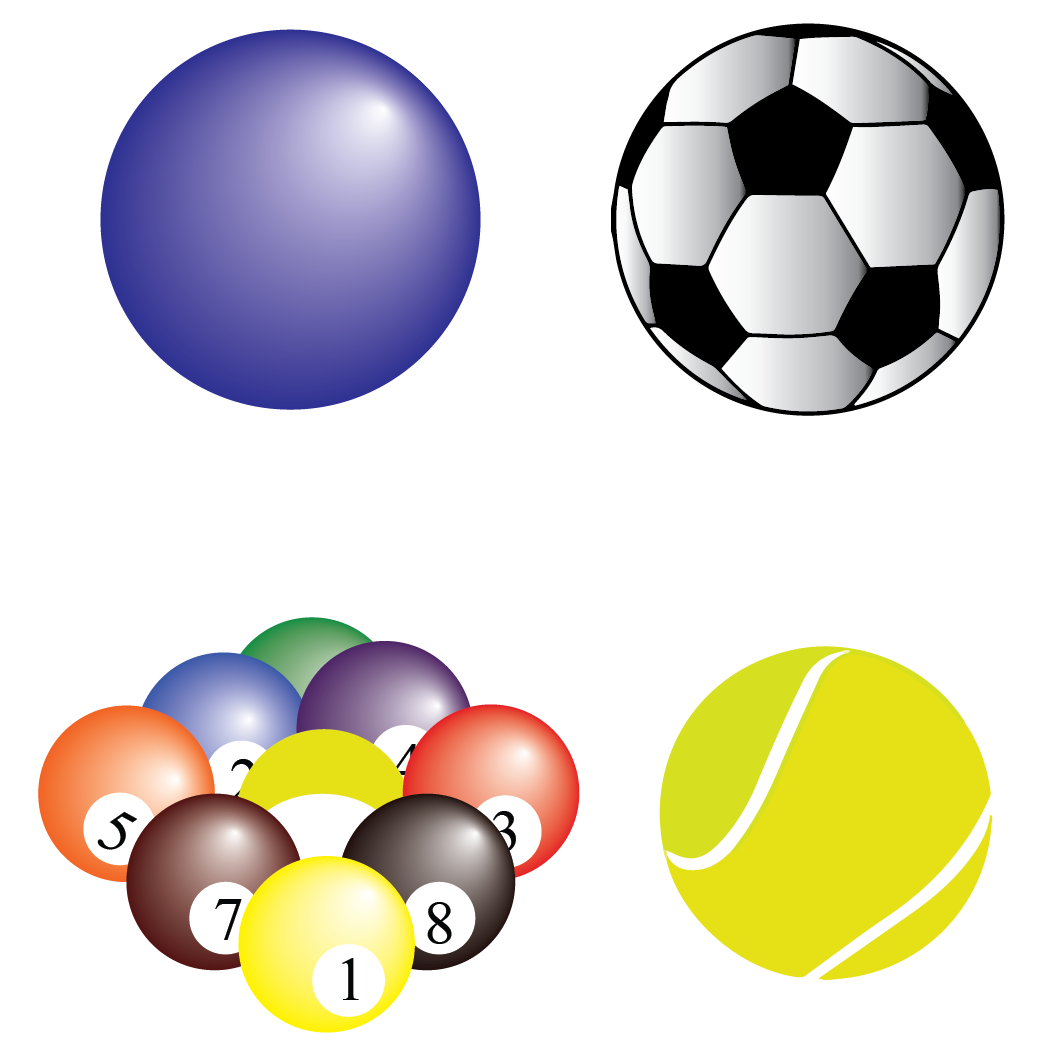Do you like ice cream?

What type of ice cream do you prefer?

If you observe an ice cream cone, you may be able to notice that it has a distinct shape too.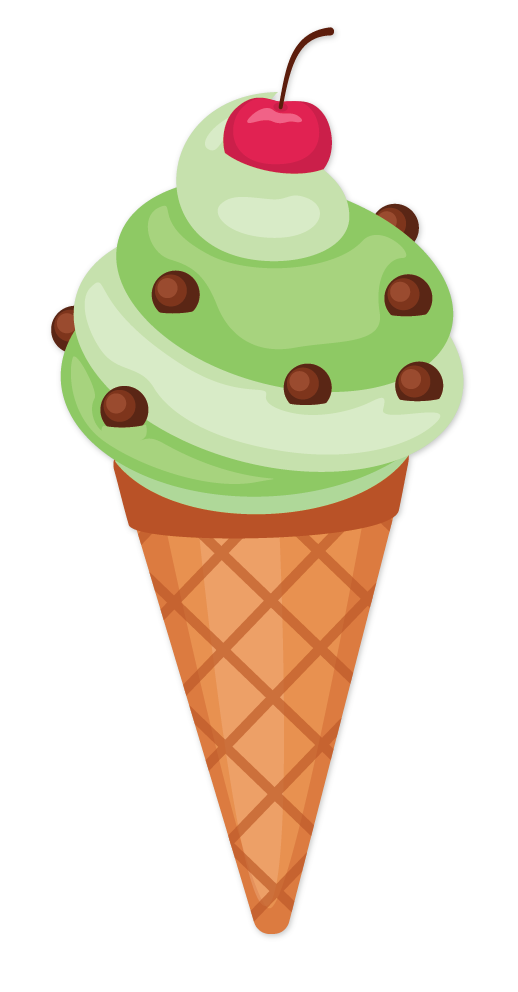What is this shape?

You are right! It is a cone.

### Cone

A cone is a three-dimensional shape that is derived from a triangle.

You can observe that the following objects are all conical in shape.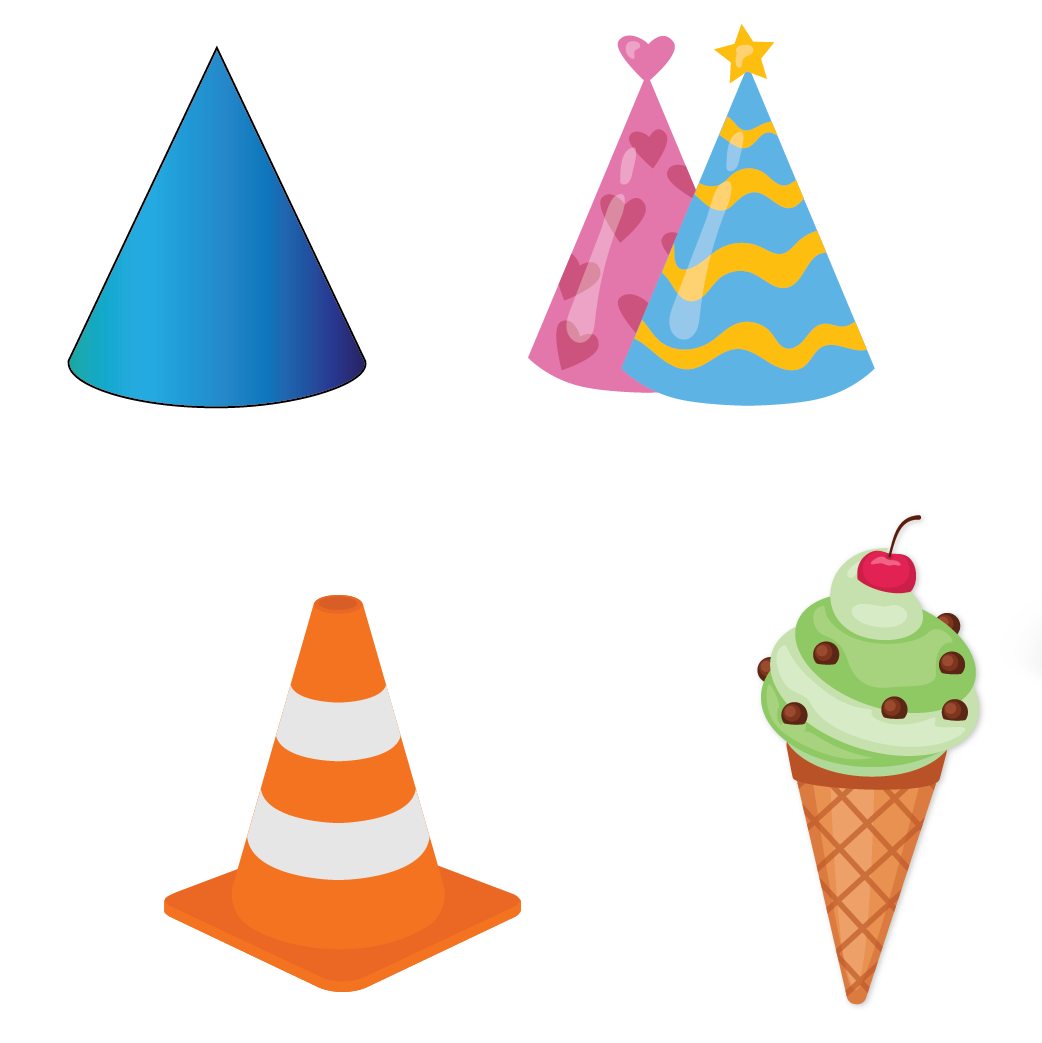Have you ever played with a Rubric's square?

The Rubric's square shown below has 6 faces with 6 colors each.

We shuffle and arrange until we get the same color on each face.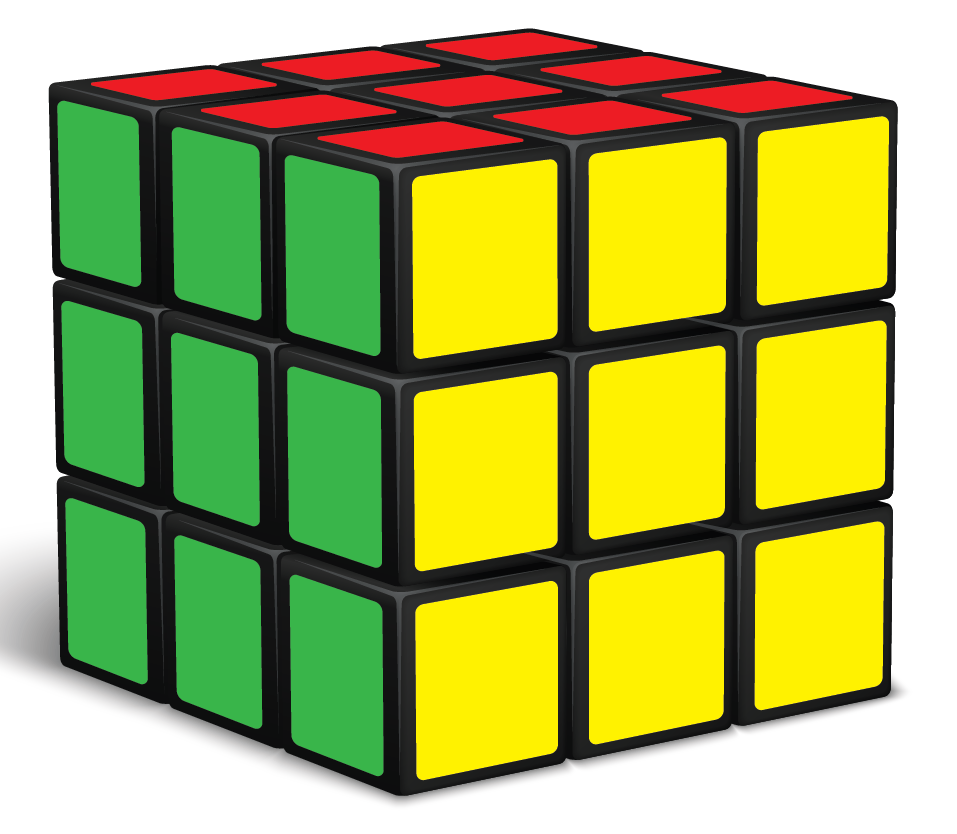Have you noticed its shape?

What is the shape of this Rubric's square?

It is a cube.

### Cube

A cube is a three-dimensional solid object with 6 square faces.

Look at the figures shown below.

Are they all cubes?

Do you notice a pencil box among the figures?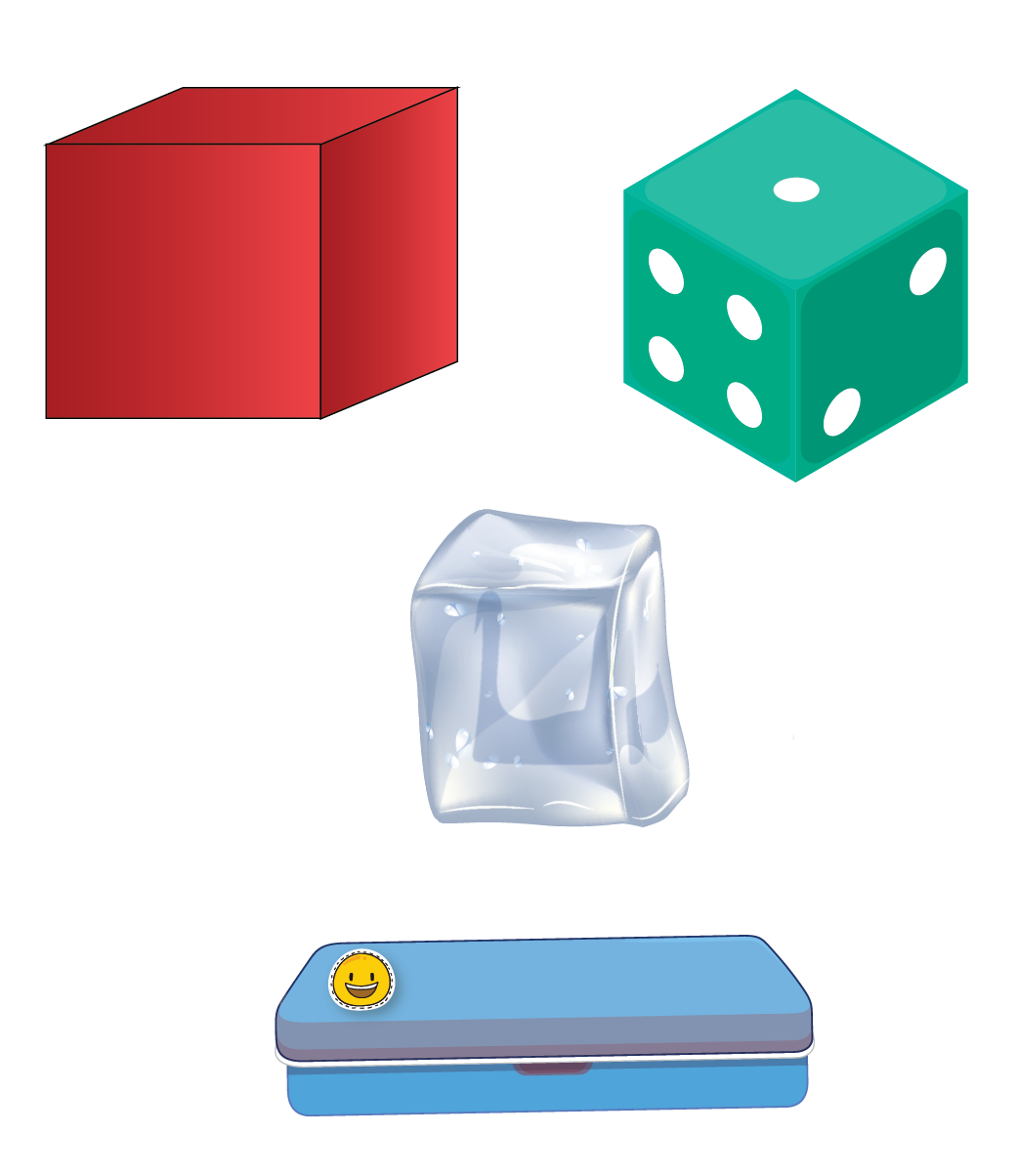Would you agree that the pencil box has the shape of a cube?

No, the shape of the pencil box is not a cube.

It is a cuboid.

### Cuboid

A cuboid is a three-dimensional solid figure which has six faces like a cube but it is derived from a rectangle.

The figures shown below are examples of cuboids.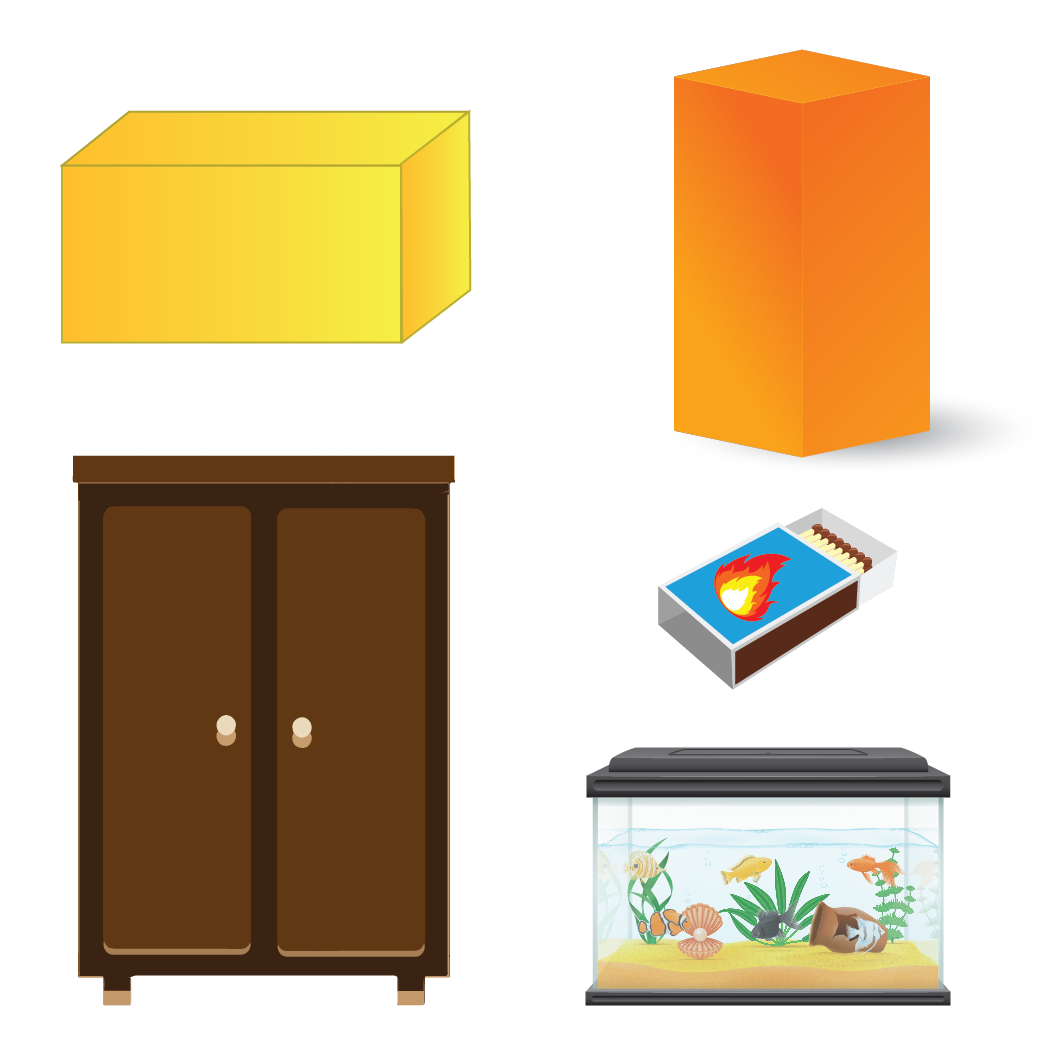Likewise, there are many different three-dimensional shapes present in geometry.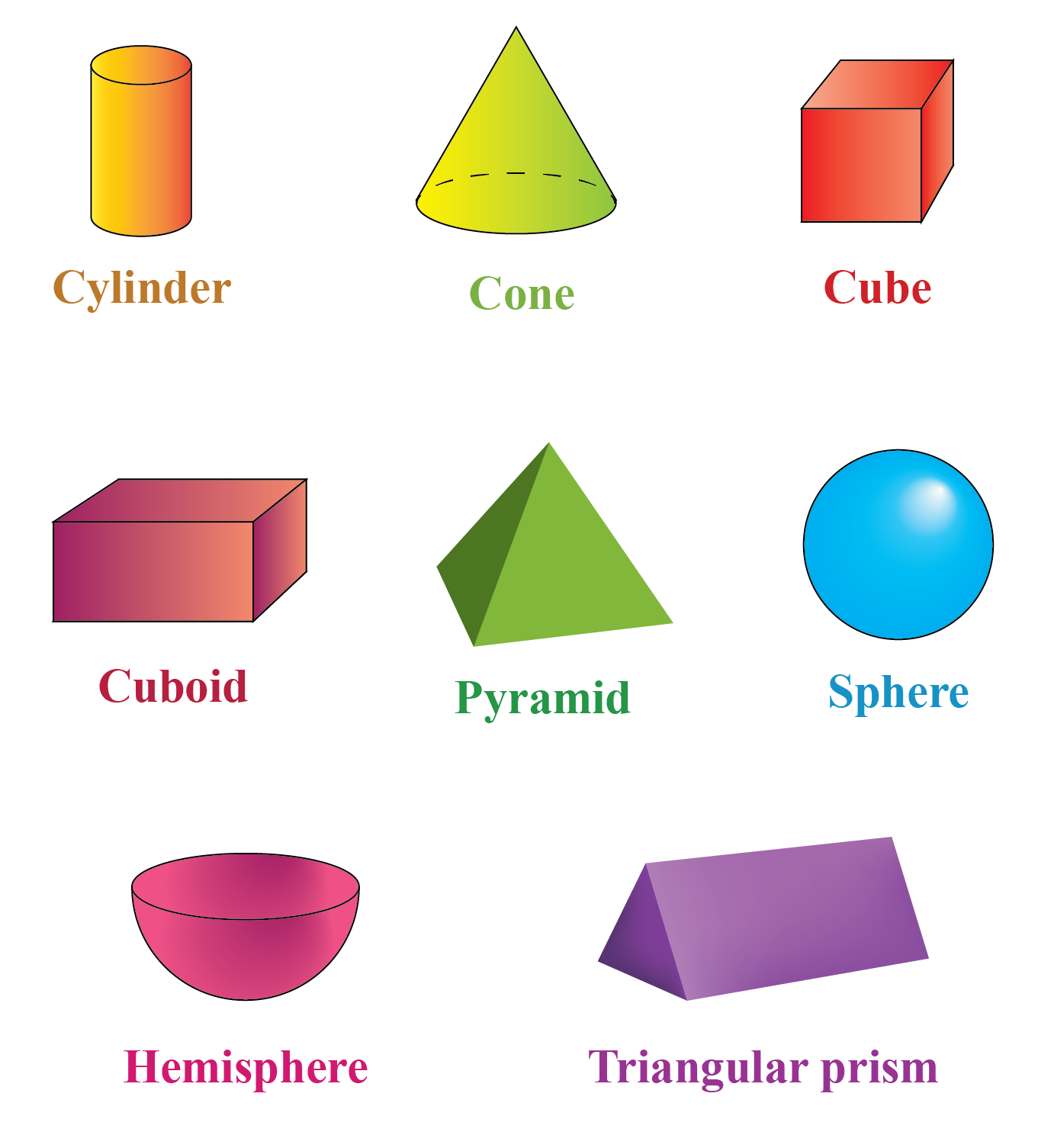Look around you and identify various shapes with three dimensions.

Help your child score higher with Cuemath’s proprietary FREE Diagnostic Test. Get access to detailed reports, customised learning plans and a FREE counselling session. Attempt the test now.

## Solved Examples on Shapes

 Example 1

Reena invited her friends for her birthday party.

She distributed birthday caps to all her friends.

Identify the shape of their birthday caps.Solution:

Their birthday caps resemble the shape of a cone.

 Example 2

Sonu ordered a sandwich at a restaurant.

He requested the waiter to cut the sandwich into two pieces.

What was the shape of those two pieces?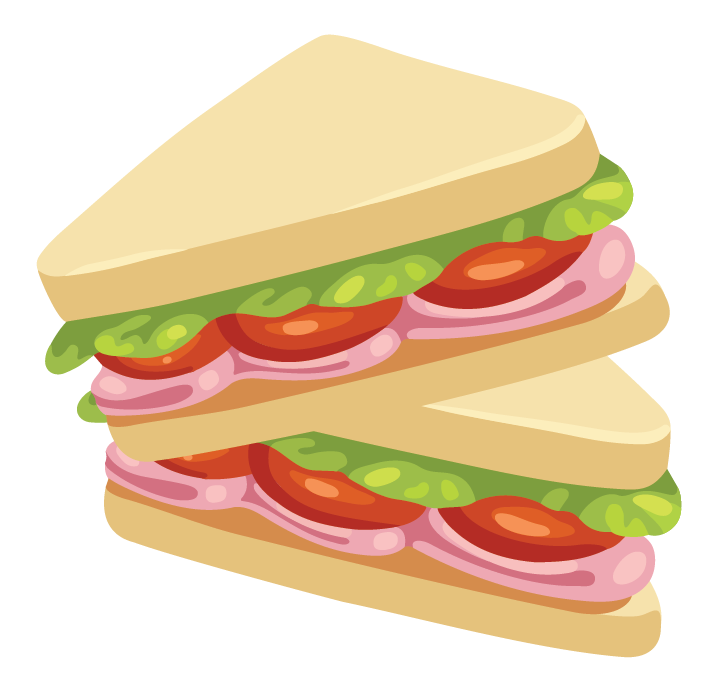Solution:

The two pieces of the sandwich were cut in the shape of a triangle.

 Example 3

He bought building blocks.

He wants to pack his gift in a box.

Help Alex find the right box to place the gift.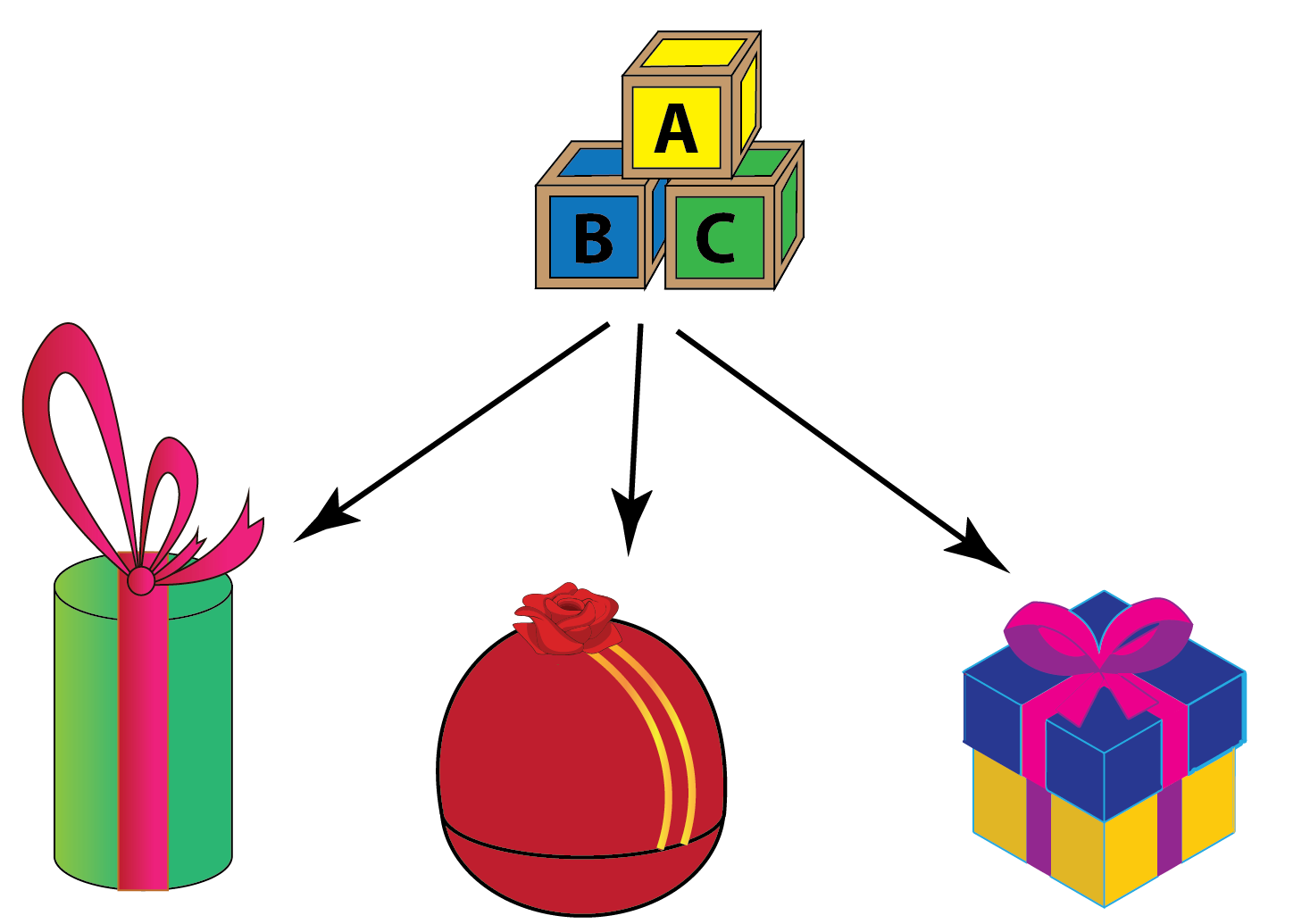Solution:

The shapes of the gift boxes are cylinder, cube and sphere.

The building blocks are in the form of a cube.

Therefore, the box shaped like a cube is the right shape to pack the building blocks.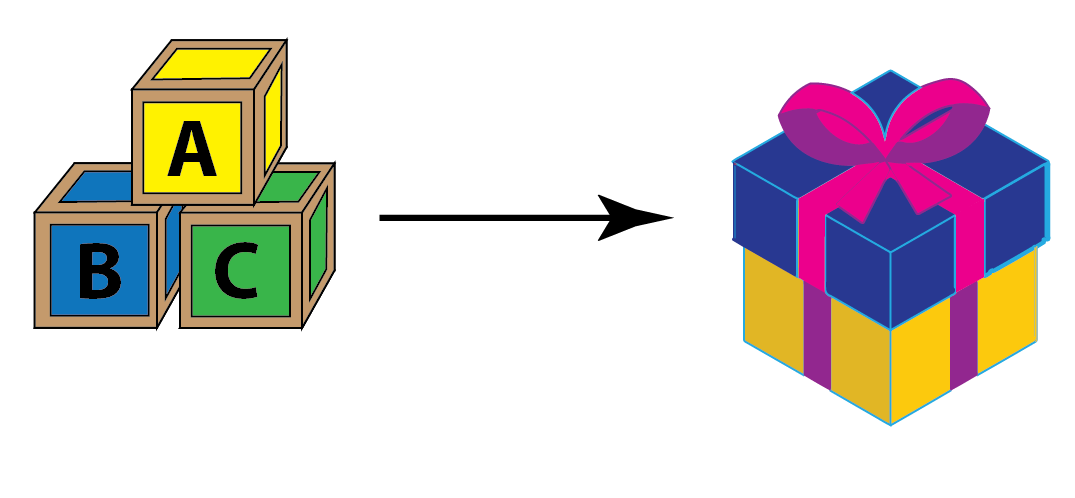Thus, Cube is the right shape.
 Example 4

How many sides does a football have?Solution:

Football is in the shape of a sphere.

Thus, it does not have any sides.

 Thus, there are zero sides in a football.

CLUEless in Math? Check out how CUEMATH Teachers will explain Geometric Shapes to your kid using interactive simulations & worksheets so they never have to memorise anything in Math again!

Explore Cuemath Live, Interactive & Personalised Online Classes to make your kid a Math Expert. Book a FREE trial class today!

## Practice Questions on Shapes

Here are a few activities for you to practice.Challenging Questions

1. How many triangles are there in this figure?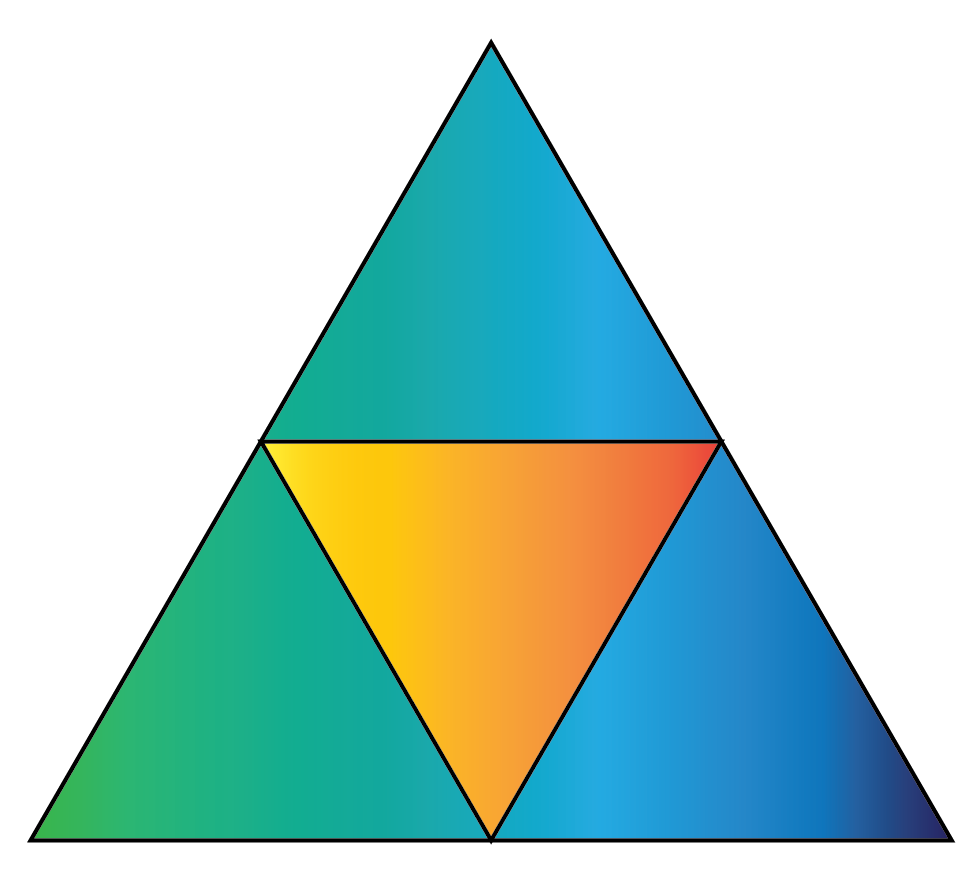IMO (International Maths Olympiad) is a competitive exam in Mathematics conducted annually for school students. It encourages children to develop their math solving skills from a competition perspective.

## 1. What are the basic geometric shapes?

The basic geometric shapes have two dimensions. They are:

• Circle

• Square

• Triangle

• Rectangle

• Oval

## 2. What are the 16 basic shapes?

The 16 basic shapes are: Triangle, Square, Rectangle, Kite, Rhombus, Trapezium, Pentagon, Hexagon, Nonagon, Decagon, Dodecagon, Hexadecagon, Star, Oval, Ellipse and Circle.

## 3. What are all the shapes in geometry?

There are many different shapes in geometry which are open or closed, two dimensional or three dimensional.

## 4. What are the 8 basic shapes?

The eight basic shapes are: circle, triangle, rectangle, square, kite, star, heart and oval.

More Important Topics
Numbers
Algebra
Geometry
Measurement
Money
Data
Trigonometry
Calculus